UR Calculus Placement Self-Assessment Exam
GOAL: The purpose of this exam is to help you decide which calculus class to take first. If you have taken a class in calculus but are not sure whether to enroll in MATH 211 (Calculus I) or MATH 212 (Calculus II), this is the place to start.

NOTE: We ask for an email address in order to send you a copy of your exam, which you may want to share with your advisor. However, the results are not directly used by the department. Ultimately you will are free to enroll in whichever course you think is best for your needs. Note that this exam can not be used to obtain course credit for Calculus I.

PREPARATION: It is a good idea to review Calculus I before the exam. The topics of the exam are:

1) Function properties
2) Finding derivatives using derivative rules, including power functions, exponential functions, ln x, sin x, cos x, tan x, sec x, csc x, cot x
3) Calculating tangent slopes
4) Finding tangent lines
5) Understanding what the derivative means
6) Limits (including L’Hopitals rule)
7) Calculating basic antiderivatives
8) Interpreting graphs of functions and derivatives

ADDITIONAL RESOURCES: Do not use a calculator, textbook, notes or any other aid while working on the exams. You may find it useful to have scrap paper and a pencil available.

TIME LIMIT: Use as much time as you need.

RESULTS: After you have submitted the exam for grading, you will see your result on each question. Your total score, along with the appropriate placement advice for that score, will be at the bottom of the page.

01-05 points: You appear to have substantial gaps in your Calculus knowledge. We recommend
that you enroll in Math 211.
06-10 points: You appear to have some major gaps in your Calculus knowledge. We do not
recommend enrolling in Math 212 unless you plan to spend substantial time
studying Calculus 1 topics before the semester
11-15 points: You appear to have a solid base for your Calculus knowledge; you may just need
to fill some minor gaps before taking Calculus 2. We recommend spending time
studying topics missed on the assessment exam and enrolling in Math 212.
16-20 points: You are very well prepared for Calculus 2. We recommend enrolling in Math 212.

QUESTIONS: Contact Dr. Hoke at khoke@richmond.edu

Question 1 *
1 point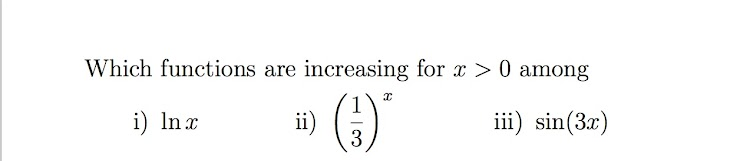Question 2 *
1 point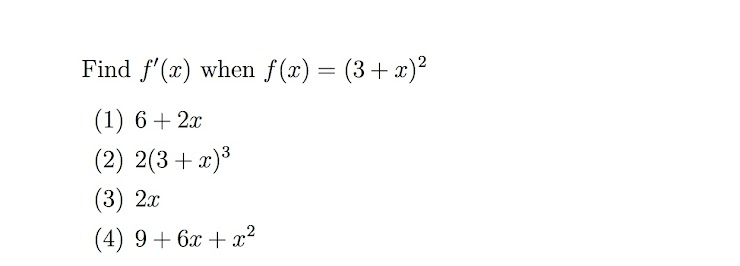Question 3 *
1 point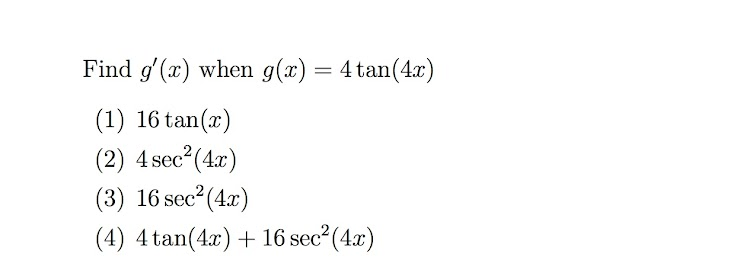Question 4 *
1 point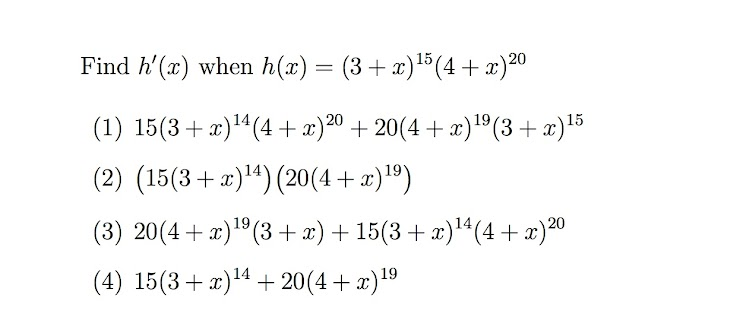Question 5 *
1 point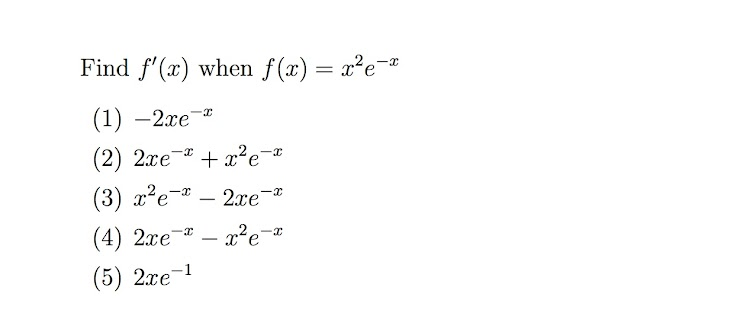Question 6 *
1 point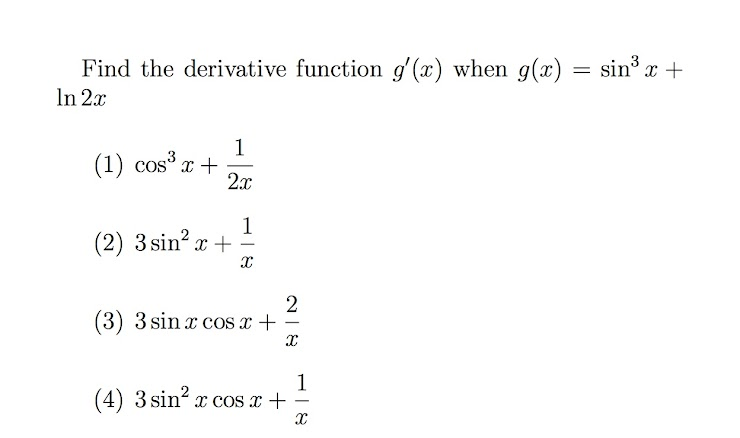Question 7 *
1 point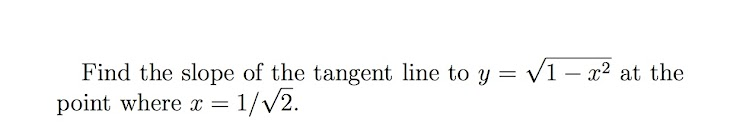Question 8 *
1 point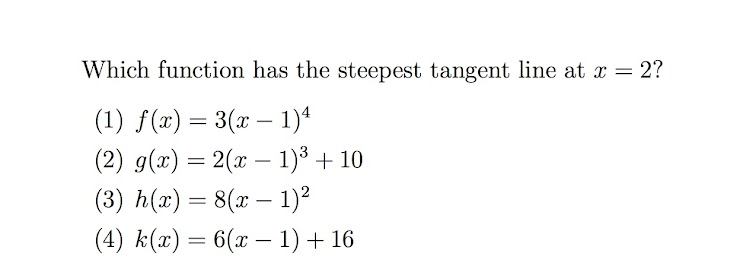Question 9 *
1 point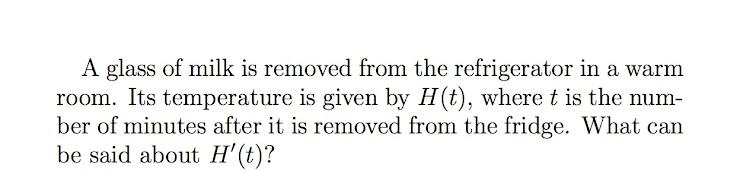Question 10 *
1 point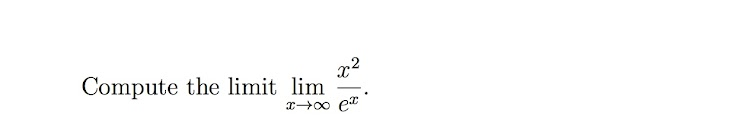Question 11 *
1 point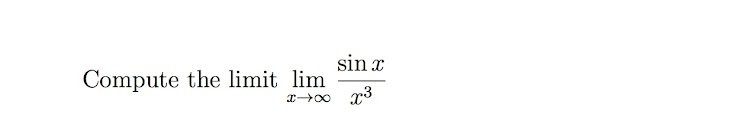Question 12 *
1 point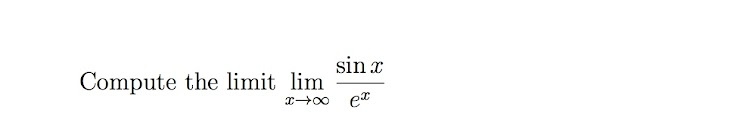Question 13 *
1 point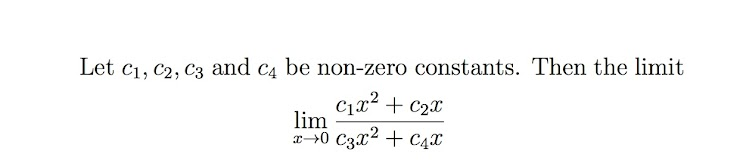Question 14 *
1 point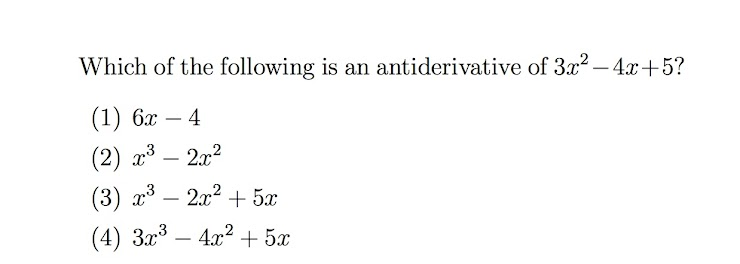Question 15 *
1 point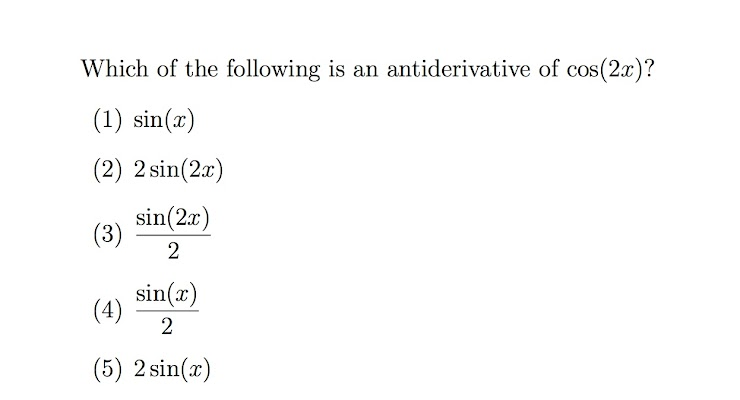Question 16 *
1 point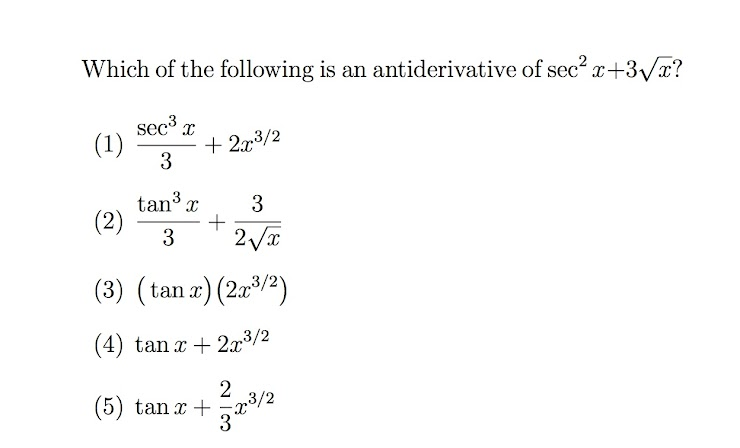Question 17
1 point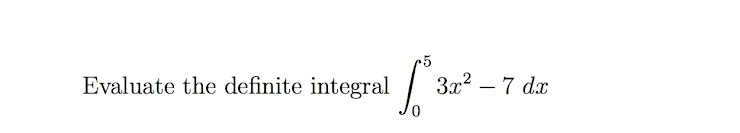Particle plot
The remaining questions refer to the graph below. It represents the velocity (not position) of two particles moving along a straight line. Both particles start at exactly the same position at time t = 0. Positive velocity indicates a particle moving to the right, while negative velocity means it is moving to the left.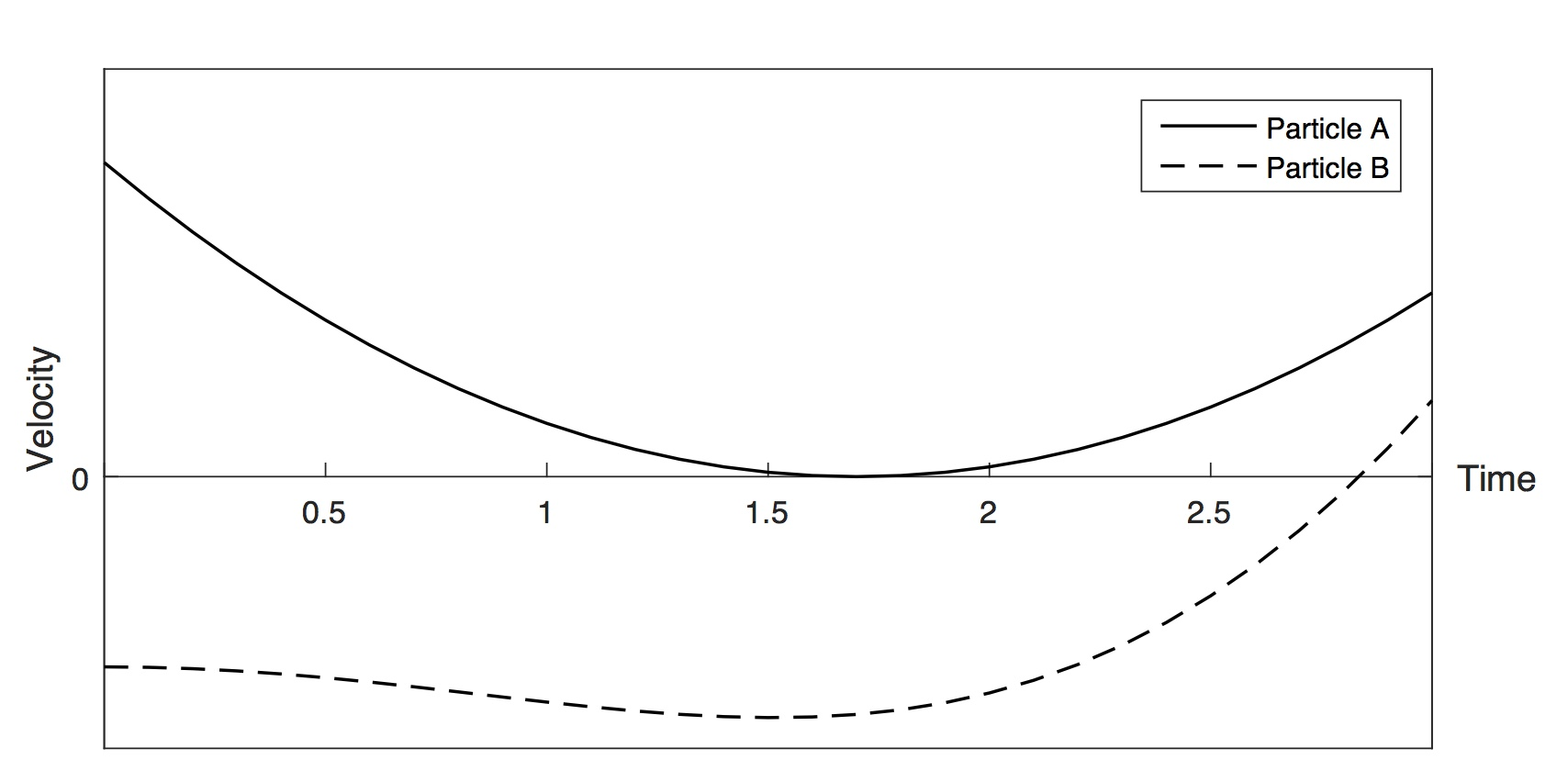Question 18 *
1 point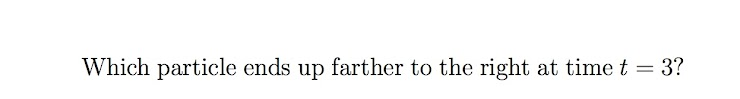Question 19 *
1 point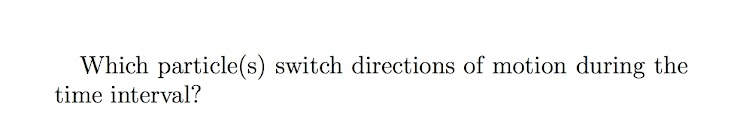Question 20 *
1 point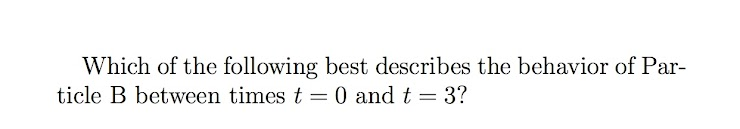A copy of your responses will be emailed to the address you provided.